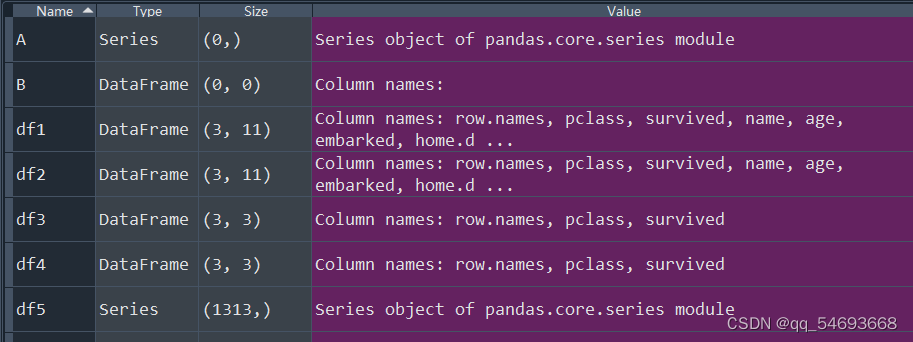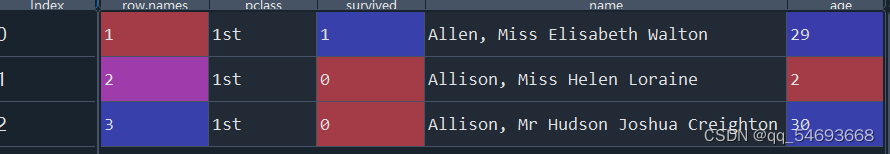﻿• 价格透明
• 信息保密
• 进度掌控
• 售后无忧# 【Day1】Python进行excel切片的方法

Excel是一个强大的表格制作工具。然而我们却很难同时修改成百上千个表格，即使是重复单一相同的操作。因此，为了节省企业运营部门处理电子表单的时间成本，本文开辟一些实现excel自动化的python代码。

进行自动化需要一些前置操作，需要：1. 安装python的pandas和openpyxl库（pip或conda安装均可，环境配置按此不表）；2. 推荐安装spyder作为python编译器（相比pycharm或anaconda，spyder有强大的数据可视化模块。同样使用pip或conda等安装均可）。

``````import pandas as pd
import openpyxl
#import路径中的csv文件
#为了方便spyder可视化，定义列表和数组
A = pd.Series()
B = pd.DataFrame()
titanic.to_excel(r'C:\Users\86186\Desktop\泰坦尼克号.xlsx')
titanic.info()``````

至此，我们成功将csv文件导入成为dataframe格式。

接下来我们正式开始。为了灵活剪切、复制和移动excel表格中的某些行或某些列，我们需要学习一个最基础却最重要的操作：数据切片。在此引入两个函数，loc和iloc，都可以切开某行至某行（某列至某列）的数据，但二者有轻微差别。我们知道在python中，导入dataframe时会自动为数据赋予以0开始的序号。而iloc和loc区别就在于，loc按照dataframe行列序号切（见df1）而iloc是按字符切（见df2，df3）。

``````#loc与iloc的区别
df1 = titanic.loc[0:2,:]
df2 = titanic.iloc[0:3,:]
df3 = titanic.loc[0:2,'row.names':'survived']
df4 = titanic.iloc[0:3,0:3]``````

我们还可以单独切某一列

``````#单独切某一列
df5 = titanic['survived']
df6 = titanic.loc[:,'survived']``````

有时，我们想按照内容切出具体的某一行或某一列，就需要配合is in函数：

``````#根据某一列拆分数据集
df7 = titanic[titanic['survived'].isin()]
df8 = titanic[titanic['survived']==1]
df9 = titanic.query('survived==1')``````

在切片等操作完成后，不要忘记reset dataframe的序号，下面展示三种不同的方式（drop可不写，inplace代表序号替换）：

``````df10 = df7.reset_index(drop = True)
df7.reset_index(inplace = True,drop = True)
df8.reset_index(drop = True)``````

除了用loc切片之外，相反地，我们还可以使用concat函数将数据整合起来：

``````total = pd.concat([df7,df8,df9,df10],axis=0)#0代表合并行
total1 = pd.concat([df7,df10],axis=1)#1代表合并列``````

到这里，我们今天的内容就结束了。值得一提的是，为什么推荐大家使用Spyder，是因为它的数据可视化窗口真的很方便。以本文为例，我们可以在工作台中随时检查define的函数维度、数据格式等：点开某一栏，还可以查看切片后某一片段的具体数值：这是其他编译器所没有的优势。今天就到这里！### 低价透明### 金牌服务### 信息保密